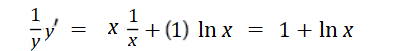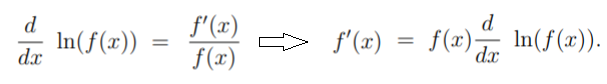# Logarithmic Differentiation (Logarithmic Derivative)

## Overview

The logarithmic derivative is where you find the logarithm of a function and then take the derivative. It is often used when it’s easier to find the derivative of the function’s logarithm, rather than the function itself.

One way to define Logarithmic differentiation is where you take the natural logarithm* of both sides before finding the derivative. This can be a useful technique for complicated functions where you can’t easily find the derivative using the usual rules of differentiation.

*The natural logarithm of a number is its logarithm to the base of e.

## When to Use Logarithmic Differentiation

In general, functions of the form y = [f(x)]g(x) work best for logarithmic differentiation, where:

• The functions f(x) and g(x) are differentiable functions of x.
• It’s easier to differentiate the natural logarithm rather than the function itself.

The technique can also be used to simplify finding derivatives for complicated functions involving powers, products, and quotients (division).

Although the process sounds simple (and it is, compared to trying to differentiate the “usual” way), it’s really an advanced differentiation technique. You do need to have some knowledge of logarithmic properties and differentiation rules. You should also have some pretty strong algebra skills and be familiar with implicit differentiation.

For example, these logarithm rules will come in handy:

1. logb(x · y) = logb(x) + logb(y)
2. logb(x / y) = logb(x) – logb(y)
3. log(ba) = a log b

## General Evaluation Steps & Simple Example

Follow these general steps to find a derivative using logarithmic differentiation:

Step 1: Take the natural log of both sides:
ln y = ln u

Step 2: Use the logarithm rules to remove as many exponents, products, and quotients as possible. In addition, use the following properties of the natural logarithm, if applicable:

1. ln (1) = 0,
2. ln (e) = 1,
3. ln ex = x.

Step 3: Differentiate both sides of the equation.

Step 4: Simplify.

Example question: Differentiate y = xx using logarithmic differentiation:

Step 1: Apply the natural log to both sides:
ln y = ln xx

Step 2: Use the laws to simplify as much as possible:
ln y = ln xxx ln x

Step 3: Differentiate both sides. For this particular function, use the chain rule on the left side and the product rule on the right:Step 4: Multiply both sides by y:
y′ = y (1 + ln x) = xx(1 + ln x)

## A More Complicated Example with the Chain Rule

If f is a real-valued function, differentiable and f(x) ≠ 0, then the logarithmic derivative of f is evaluated with the chain rule: f′(x) / f(x). In notation, that’s:Using similar logic, the log derivative of fg is the log derivative of f + log derivative of g.

Example problem: Find the logarithmic derivative of ln(√sin x)

Step 1: Convert the radical into an exponent:Step 2: Bring the ½ out in front (because of logarithm rule #3, above):Step 3: Use the chain rule (defined above as lv(f(x))′ = f&prime(x) = f(x)) to get:As the cotangent function is the ratio cos(x) / sin(x), this can be rewritten as ½ cot(x).

## Complex-Valued Logarithmic Differentiation

The logarithmic derivative becomes more complicated with complex-valued functions. Although you can still use the chain rule f′(z) / f(z).

Example: The logarithmic derivative of an n-degree polynomial function:Is given by (Anderson & Eiderman, 2006):For complex-valued functions like this one, there aren’t actually any logarithms involved (Montgomery, 2014).

## Logarithmic Derivative vs. Logarithmic Function

A logarithmic derivative is different from the logarithm function. With logarithmic differentiation, you aren’t actually differentiating the logarithmic function f(x) = ln(x). Instead, you’re applying logarithms to nonlogarithmic functions.

• The logarithm function is a very specific function f(x) = logbx—the inverse of the exponential function.
• The logarithmic derivative can be any function that you find the log for, and take the derivative. For example:

## References

Anderson, J. & Eiderman, V. Cauchy transforms of point masses: The log. derivative of polynomials. Annals of Mathematics, 163 (2006), 1057–1076.
Bird, J. (2017). Higher Engineering Mathematics. Routledge.
Ghosh, J. (2004). How to Learn Calculus of One Variable: v. 1. New Age International Publisher.
Larson, R. (2011). Calculus I with Precalculus. Cengage Learning.
Montgomery, H. (2014). Early Fourier Analysis. American Mathematical Society.
Rhode, U et al. (2012). Introduction to Differential Calculus: Systematic Studies with Engineering Applications for Beginners 1st Edition. Wiley.

CITE THIS AS:
Stephanie Glen. "Logarithmic Differentiation (Logarithmic Derivative)" From StatisticsHowTo.com: Elementary Statistics for the rest of us! https://www.statisticshowto.com/logarithmic-differentiation/